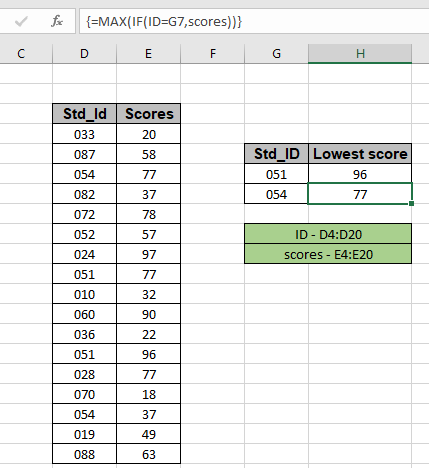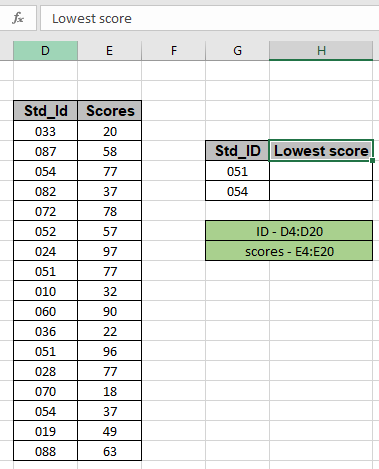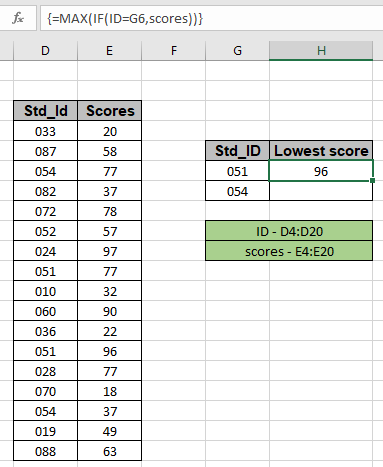How to Get Max If Satisfies Criteria in ExcelIn this article, we will learn about how to get the maximum value if condition stands True in Excel.

Scenario:

In simple words, when working with long data sheets, sometimes we need to extract the maximum value only if the condition stands true like finding the joining date by Employee code.

Generic Formula

{ = MAX ( IF (range = criteria, values)) }

range = criteria : condition on the array.

Values : corresponding array where Maximum value is to be found.

Note:

Do not use curly braces manually, use Ctrl + Shift + Enter instead of just Enter after completion of formula as this is an array formula.

Example:

Here we have expiry date of products and we need to find the earliest expiry date for the specific products.Use the formula:

{ = MAX ( IF ( ID =G7 , scores ) ) }

Explanation:

• ID = G7 matches all student ID with the value in G7 cell.
• The IF function returns the scores corresponding to the matched ID values as shown below.

= MAX ( { FALSE ; FALSE ; FALSE ; FALSE ; FALSE ; FALSE ; FALSE ; 77 ; FALSE ; FALSE ; FALSE ; 96 ; FALSE ; FALSE ; FALSE ; FALSE ; FALSE } )

• MAX function finds the maximum score out of the returned array which is our result.As you can see the formula returns maximum score gained by std_ID 051. Now copy the formula to the next cell to get the result for the next ID.As you can see the formula works fine. This is an array formula so do not forget to use the Ctrl + Shift + Enter.

Here are some observational results using the above explained formula:

1. Do not use curly braces manually, use Ctrl + Shift + Enter instead of just Enter after completion of formula as this is an array formula.
2. You can check the returned array using the F9 key.

Hope you understood how to get the Maximum value using IF function in Excel. Explore more articles on Excel logic functions here. Please feel free to state your query or feedback for the above article. If you liked our blogs, share it with your friends on Facebook. And also you can follow us on Twitter and Facebook. We would love to hear from you, do let us know how we can improve, complement or innovate our work and make it better for you. Write us at info@exceltip.com

Related Articles

IF with AND function : Implementation of logic IF function with AND function to extract results having criteria in Excel.

IF with OR function : Implementation of logic IF function with OR function to extract results having criteria in excel data.

How to use nested IF function : nested IF function operates on data having multiple criteria. The use of repeated IF function is nested IF excel formula.

SUMIFS using AND-OR logic : Get the sum of numbers having multiple criteria applied using logic AND-OR excel function.

Minimum value using IF function : Get the Minimum value using the excel IF function and MIN function on array data.

How to use wildcards in excel : Count cells matching phrases using the wildcards in excel

Popular Articles

50 Excel Shortcut to Increase Your Productivity : Get faster at your task. These 50 shortcuts will make you work even faster on Excel.

The VLOOKUP Function in Excel : This is one of the most used and popular functions of excel that is used to lookup value from different ranges and sheets.

COUNTIF in Excel 2016 : Count values with conditions using this amazing function. You don't need to filter your data to count specific values. Countif function is essential to prepare your dashboard.

How to Use SUMIF Function in Excel : This is another dashboard essential function. This helps you sum up values on specific conditions.

Terms and Conditions of use

The applications/code on this site are distributed as is and without warranties or liability. In no event shall the owner of the copyrights, or the authors of the applications/code be liable for any loss of profit, any problems or any damage resulting from the use or evaluation of the applications/code.